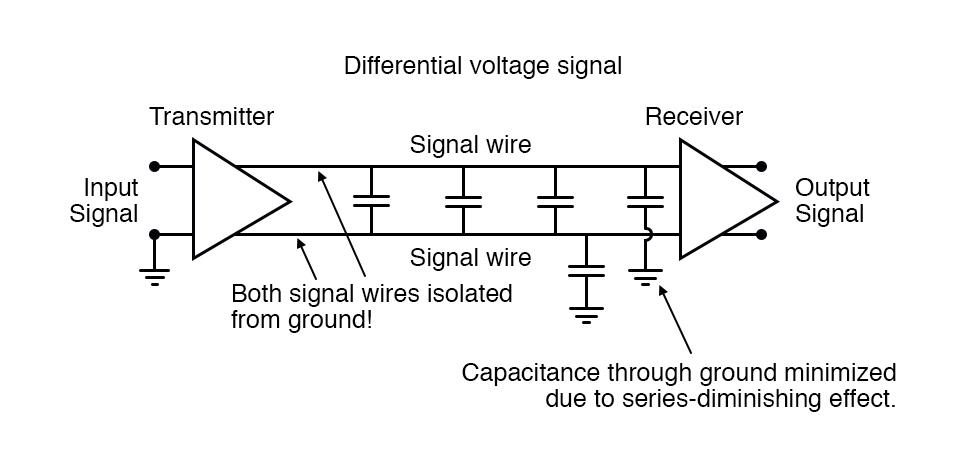## How do you avoid square wave signals?

One way to avoid this is to modulate a sine wave voltage signal with our digital data. “Modulation” means that magnitude of one signal has control over some aspect of another signal.

# Electrical Signal Types

## Chapter 14 - Digital Communication

With BogusBus, our signals were very simple and straightforward: each signal wire (1 through 5) carried a single bit of digital data, 0 Volts representing “off” and 24 Volts DC representing “on.” Because all the bits arrived at their destination simultaneously, we would call BogusBus a parallel network technology.

If we were to improve the performance of BogusBus by adding binary encoding (to the transmitter end) and decoding (to the receiver end), so that more steps of resolution were available with fewer wires, it would still be a parallel network.

If, however, we were to add a parallel-to-serial converter at the transmitter end and a serial-to-parallel converter at the receiver end, we would have something quite different.

It is primarily with the use of serial technology that we are forced to invent clever ways to transmit data bits.

Because serial data requires us to send all data bits through the same wiring channel from transmitter to receiver, it necessitates a potentially high frequency signal on the network wiring.

Consider the following illustration: a modified BogusBus system is communicating digital data in parallel, binary-encoded form. Instead of 5 discrete bits like the original BogusBus, we’re sending 8 bits from transmitter to receiver.

The A/D converter on the transmitter side generates a new output every second. That makes for 8 bits per second of data being sent to the receiver.

For example, the transmitter is bouncing between an output of 10101010 and 10101011 every update (once per second):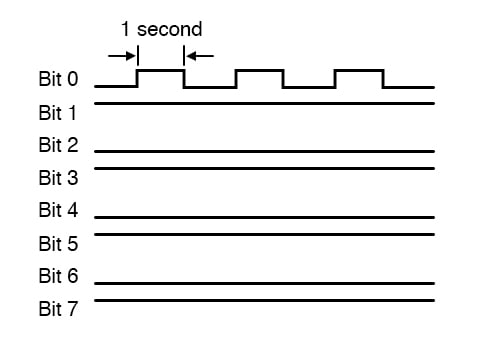Since only the least significant bit (Bit 1) is changing, the frequency on that wire (to ground) is only 1/2 Hertz. In fact, no matter what numbers are being generated by the A/D converter between updates, the frequency on any wire in this modified BogusBus network cannot exceed 1/2 Hertz, because that’s how fast the A/D updates its digital output. 1/2 Hertz is pretty slow, and should present no problems for our network wiring.

On the other hand, if we used an 8-bit serial network, all data bits must appear on the single channel in sequence. And these bits must be output by the transmitter within the 1-second window of time between A/D converter updates.

Therefore, the alternating digital output of 10101010 and 10101011 (once per second) would look something like this: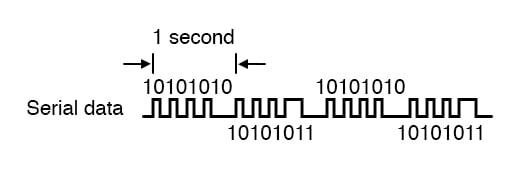The frequency of our BogusBus signal is now approximately 4 Hertz instead of 1/2 Hertz, an eightfold increase!

While 4 Hertz is still fairly slow, and does not constitute an engineering problem, you should be able to appreciate what might happen if we were transmitting 32 or 64 bits of data per update, along with the other bits necessary for parity checking and signal synchronization, at an update rate of thousands of times per second!

Serial data network frequencies start to enter the radio range, and simple wires begin to act as antennas, pairs of wires as transmission lines, with all their associated quirks due to inductive and capacitive reactances.

Data being process in a serial network communication are square waves data which binary bits of information. Square waves are peculiar things, being mathematically equivalent to an infinite series of sine waves of diminishing amplitude and increasing frequency.

A simple square wave at 10 kHz is actually “seen” by the capacitance and inductance of the network as a series of multiple sine-wave frequencies which extend into the hundreds of kHz at significant amplitudes. What we receive at the other end of a long 2-conductor network won’t look like a clean square wave anymore, even under the best of conditions!

Bandwidth

When engineers speak of network bandwidth, they’re referring to the practical frequency limit of a network medium. In serial communication, bandwidth is a product of data volume (binary bits per transmitted “word”) and data speed (“words” per second).

The standard measure of network bandwidth is bits per second, or bps. An obsolete unit of bandwidth known as the baud is sometimes falsely equated with bits per second, but is actually the measure of signal level changes per second.

Many serial network standards use multiple voltage or current level changes to represent a single bit, and so for these applications bps and baud are not equivalent.

Common Ground Method

The general BogusBus design, where all bits are voltages referenced to a common “ground” connection, is the worst-case situation for high-frequency square wave data communication.

Everything will work well for short distances, where inductive and capacitive effects can be held to a minimum, but for long distances this method will surely be problematic: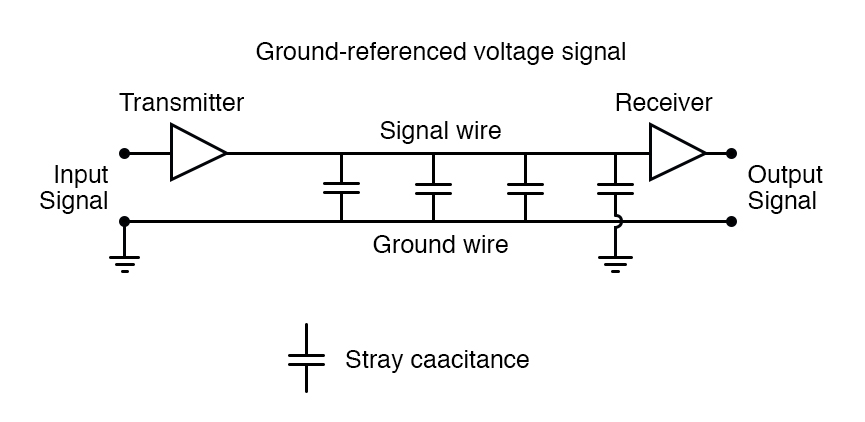Differential Voltage Method

A robust alternative to the common ground signal method is the differential voltage method, where each bit is represented by the difference of voltage between a ground-isolated pair of wires, instead of a voltage between one wire and a common ground.

This tends to limit the capacitive and inductive effects imposed upon each signal and the tendency for the signals to be corrupted due to outside electrical interference, thereby significantly improving the practical distance of a serial network: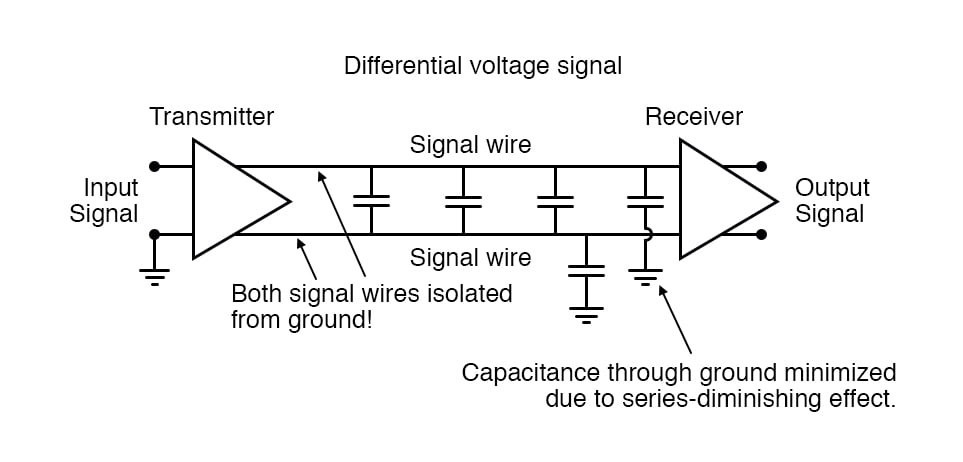The triangular amplifier symbols represent differential amplifiers, which output a voltage signal between two wires, neither one electrically common with ground. Having eliminated any relation between the voltage signal and ground, the only significant capacitance imposed on the signal voltage is that existing between the two signal wires.

Capacitance between a signal wire and a grounded conductor is of much less effect, because the capacitive path between the two signal wires via a ground connection is two capacitances in series (from signal wire #1 to ground, then from ground to signal wire #2), and series capacitance values are always less than any of the individual capacitances.

Furthermore, any “noise” voltage induced between the signal wires and earth ground by an external source will be ignored, because that noise voltage will likely be induced on both signal wires in equal measure, and the receiving amplifier only responds to the differential voltage between the two signal wires, rather than the voltage between any one of them and earth ground.

RS-232C is a prime example of a ground-referenced serial network, while RS-422A is a prime example of a differential voltage serial network. RS-232C finds popular application in office environments where there is little electrical interference and wiring distances are short.

RS-422A is more widely used in industrial applications where longer wiring distances and greater potential for electrical interference from AC power wiring exists.

However, a large part of the problem with digital network signals is the square-wave nature of such voltages, as was previously mentioned.

If only we could avoid square waves all together, we could avoid many of their inherent difficulties in long, high-frequency networks. One way of doing this is to modulate a sine wave voltage signal with our digital data.

“Modulation” means that magnitude of one signal has control over some aspect of another signal. Radio technology has incorporated modulation for decades now, in allowing an audio-frequency voltage signal to control either the amplitude (AM) or frequency (FM) of a much higher frequency “carrier” voltage, which is then send to the antenna for transmission.

The frequency-modulation (FM) technique has found more use in digital networks than amplitude-modulation (AM), except that its referred to as Frequency Shift Keying (FSK). With simple FSK, sine waves of two distinct frequencies are used to represent the two binary states, 1 and 0: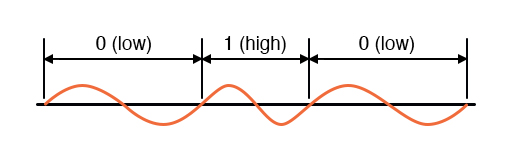Due to the practical problems of getting the low/high frequency sine waves to begin and end at the zero crossover points for any given combination of 0’s and 1’s, a variation of FSK called phase-continuous FSK is sometimes used, where the consecutive combination of a low/high frequency represents one binary state and the combination of a high/low frequency represents the other.

This also makes for a situation where each bit, whether it be 0 or 1, takes exactly the same amount of time to transmit along the network: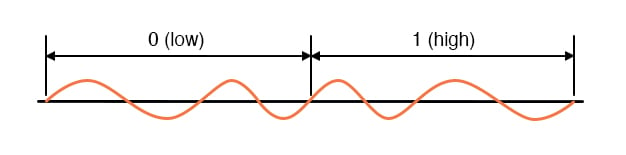With sine wave signal voltages, many of the problems encountered with square wave digital signals are minimized, although the circuitry required to modulate (and demodulate) the network signals is more complex and expensive.

RELATED WORKSHEETS:

• Share
Published under the terms and conditions of the Design Science License